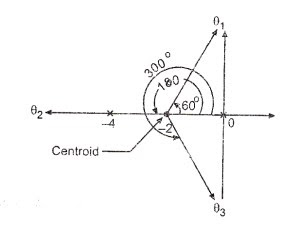# How to draw root locus graph with simple steps

We know that the stability of the given closed loop system depends upon the location of the roots of the characteristics equation. That is the location of the closed loop poles. If we change some parameter of a system, then the location of closed loop pole changes in ‘s’ plane. The study of this locus (of moving pole in ‘s’ plane) because of variation of any parameter of the system is very important while designing any closed loop system.

This movement of poles in ‘s’ plane is called as ‘Root Locus’.

This method was invented by W.R. Evans in 1948.

Root Locus is a simple graphical method for determining the roots of the characteristic equation. It can be drawn by varying the parameter (generally gain of the system but there are also other parameters that can be varied) from zero to infinity.

### Root Locus Method with step by step solution

General steps for drawing the Root Locus of the given system:

1. Determine the open loop poles, zeros and a number of branches from given G(s)H(s).

2. Draw the pole-zero plot and determine the region of real axis for which the root locus exists. Also, determine the number of breakaway points (This will be explained while solving the problems).

3. Calculate the angle of asymptotes.

4. Determine the centroid.

5. Calculate the breakaway points (if any).

6. Calculate the intersection point of root locus with the imaginary axis.

7. Calculate the angle of departure or angle of arrivals if any.

8. From above steps draw the overall sketch of the root locus.

9. Predict the stability and performance of the given system by the root locus.

## Solved Problem on Root Locus:

Question: For a unity feedback system, G(s) = K/[s(s+4)(s+2)]. Sketch the nature of root locus showing all details on it. Comment on the stability of the system.

Solution:

Given system is unity feedback system. Therefore H(s) = 1.

Therefore G(s) H(s) = K/[s(s+4)(s+2)].

Step 1:

Poles = 0, -4, -2. Therefore P=3.

Zeros = there are no zeros. Z=0.

So all (P-Z=3) branches terminate at infinity.

Step 2: Pole-zero plot and sections of the real axis.

The pole-zero plot of the system is shown in the figure below. Here RL denotes Root Locus existence region and NRL denotes the non-existence region of root locus.These sections of real axis identified as a part of the root locus as to the right sum of poles and zeros is odd for those sections.

Step 3: Angle of asymptotes

'A line to which root locus touches at infinity is called asymptotes.'

Number of asymptotes = P-Z = 3. Therefore 3 asymptotes are approaching to infinity.Step 4: Centroid or Centre of asymptotes.

Asymptote touches real axis at a point called centroid.

Branches will approach infinity along these lines which are asymptotes.Step 5: To find a breakaway point, we have the characteristic equation as,As there is no root locus between -2 to -4, -3.15 can not be a breakaway point. it also can be confirmed by calculating 'K' for s = -3.15. It will be negative that confirms s = -3.15 is not a breakaway point.

For s = -3.15, K = -3.079 (Substituting in equation for K). But as there has to be breakaway point between'0'and '-2’, s = - 0.845 is a valid breakaway point.

For s = -0.845 K = +3.079. As K is positive s = - 0.845 is valid breakaway point.

Step 6: Intersection point with the imaginary axis.

Characteristic equation

s^3+6s^2 +8s+K = 0

Routh's array:Intersection of root locus with imaginary axis is at ±j2.828 and corresponding value of K(marginal) = 48.

Step 7: As there are no complex conjugate poles or zeros, no angles of departures or arrivals are required to be calculated.

Step 8: The complete root locus is as shown in the figure below.Step 9: Prediction of stability:

For 0 < K < 48, all the roots are in left half of s-plane hence the system is absolutely stable.

For K(marginal) = +48, a pair of dominant roots on imaginary axis with remaining root in left half. So the system is marginally stable oscillating at 2.82 rad/sec. For 48 < K < 8, dominant roots are located in right half of s-plane hence system is unstable.

Stability is predicted by locations of dominant roots. Dominant roots are those which are located closest to the imaginary axis.

### MATLAB Program for Root Locus Method:

`%AIM: To plot the root locus of given transfer function%Created by : myclassbook.orgclc;clear all;close all;s=tf('s');k=1;G=k/(s*(s+4)*(s+2))rlocus(G)`

### You may also like:

1.Thank you very much! This was very helpful.

2.If angle of departures or arrival calculation also explain through an exemple, then it was more effective. Though, thanks for your explaination.

3.4.5.it is very helpful .. thanks a lot :D

6.7.Malek alwerfallyJuly 8, 2018 at 9:47 PM

Draw root locus?
10s+k/s^2(s+6)
daming ratio of dominant poles is 0.6
8.9.